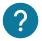# What is a string? How to do operations? How to convert a string's number into an integer ?

## Introduction

String variables allow you to store strings in your programs. In a string you can store any kind of Ascii character. Thanks to this type of variable you can write messages for example.

Here is how to declare a string:

``String string_variable = "Arduino Factory";  ``### What is the ASCII table?

The ASCII table is the set of characters you can use in your string. Here is a table that summarizes all these characters:In this table we will only use the char column. Indeed, in this column, you will find all the characters that you can put in your variable.### What is the difference between a char and a string?

The char and string variables are quite similar: they both allow to store strings. However, char variables are more used to store a character or to access a character in a string. Whereas a string variable is more used to store several characters and to do operations on them, like concatenate them for example.

We will see at the end of this course how to convert a string to a char.

## Operation on string's variable

We will now see different operations that we can do on strings:

A) Find the place of an element in a string

Here is how to know the place of an element in a string:

``int place = your_string.indexOf("your element"); // The place of the element choosen is store in place variable``

We can give you an example to better understand its use:

``````String string_site = "Arduino Factory";
void setup() {
Serial.begin(9600);
Serial.print("the position of the element r is:");
Serial.println(string_site.indexOf("r"));// return 1 because r is at the position 1 of our string
}

void loop() {
}``````

B) The size of your string

We will learn know how to find the size of your string:

``your_string.length();``

Here is a detailed example :

``````String string_site = "Arduino Factory";
void setup() {
Serial.begin(9600);
Serial.print("The size of your string is:");
Serial.println(string_site.length());// return 15
}

void loop() {
}``````

C) Compare two strings

We will now see how to compare two strings together:

``my_string_1.compareTo(my_string_2);``

Here are the possible returns of this function:

• The number 0 is returned if both strings are identical.
• A negative number is given if my_string_1 is placed in front of my_string_2.
• A positive number is given if my_string_2 is placed in front of my_string_1.

We can give you an example to better understand its use:

``````String string_Arduino = "Arduino";
String string_Factory = "Arduino";
void setup() {
Serial.begin(9600);
Serial.print("Are the two strings identical? :");
Serial.println(string_Arduino.compareTo(string_Factory));// return 0 because theya re identical
}

void loop() {
}``````

D) Replacing one of the elements of the string

We will now see how to replace an element of a string by an another one:

``your_string.replace("one_element_of_your_string", "the_new_element");``

We can give you an example to better understand its use:

``````String string_site = "Arduino Factory";
void setup() {
Serial.begin(9600);
string_site.replace("A", "the_new_string");
Serial.println(string_site); //We have this : the_new_stringrduino Factory
}

void loop() {
}``````

This is what we have: “the_new_stringrduino Factory”.

E) Put your string in uppercase

We will now see how to put your string in uppercase :

``your_string.toUpperCase();``

We can give you an example to better understand its use:

``````String string_site = "Arduino Factory";
void setup() {
Serial.begin(9600);
string_site.toUpperCase();
Serial.println(string_site); // it's display: ARDUINO FACTORY
}
void loop () {

}``````

F) How to put your string in lower case

We will now see how to put your string in lower case:

``votre_string.toLowerCase();``

We can give you an example to better understand its use:

``````String string_site = "Arduino Factory";
void setup() {
Serial.begin(9600);
votre_string.toLowerCase();
string_site.toLowerCase();
Serial.println(string_site); // it's display: arduino factory``````

## Convert a string to float, int or char

We will now convert a string into a variable of another type, int, float or char.

A) Converting a string into an integer

We will now see how to convert a string into an integer.

``votre_string.toInt();``

Your string must be exclusively integers numbers for this to work. Otherwise you will get an error.

We can give you an example to better understand its use:

``````String string_site = "12";
void setup() {
Serial.begin(9600);
string_site.toInt();
Serial.println(string_site); // it'sdisplay 12 as an integer
}
void loop(){
}``````

B) Converting a string into a float

We will now see how to convert a string into a decimal number (float):

``your_string.toFloat()``

Your string must be exclusively decimal numbers for this to work. Otherwise you will get an error.

We can give you an example to better understand its use:

``````String string_site = "32.8";
void setup() {
Serial.begin(9600);
string_site.toFloat();
Serial.println(string_site); //it's return 32.8 as a float
}
void loop(){
}``````

C) Converting a string to a char

We will now see how to convert a string into a char.

``your_string.charAt(0);``

We can give you an example to better understand its use:

``````String string_site = "Arduino Factory";
void setup() {
Serial.begin(9600);
string_site.charAt(0);
Serial.println(string_site); // It's return "Arduino Factory" as a char
}

void loop() {
}``````